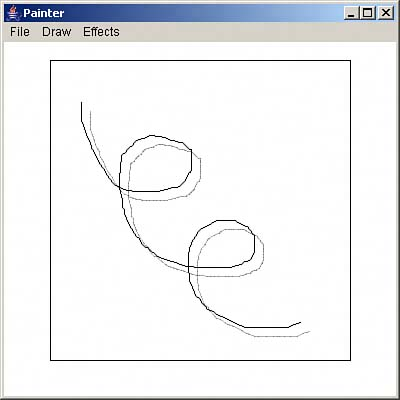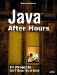Drawing Freehand

If the user has selected the Draw menu's Draw freehand item, he can use the mouse to draw freehand. As you'll recall, the successive locations of the mouse are stored in an array named dots if the user is drawing freehand, so all that's necessary is to connect those dots.

If the user wants a drop shadow, you should draw that first, then reproduce what the user has drawn with the mouse. Here's what it looks like in code:

if(draw){         Line2D.Double drawLine;         if(shadow){             paint = gImage.getPaint();             composite = gImage.getComposite();             gImage.setPaint(Color.black);             gImage.setComposite(AlphaComposite.getInstance                 (AlphaComposite.SRC_OVER, 0.3f));             for(int loop_index = 0; loop_index < dots - 1;                 loop_index++){                 if(dragging){                     drawLine = new Line2D.Double(                         dot[loop_index].x + 9,                         dot[loop_index].y + 9,                         dot[loop_index + 1].x + 9,                         dot[loop_index + 1].y + 9);                 }else{                     drawLine = new Line2D.Double(                         dot[loop_index].x - offsetX + 9,                         dot[loop_index].y - offsetY + 9,                         dot[loop_index + 1].x - offsetX + 9,                         dot[loop_index + 1].y - offsetY + 9);                 }                 gImage.draw(drawLine);             }             gImage.setPaint(paint);             gImage.setComposite(composite);         }         for(int loop_index = 0; loop_index < dots - 1;             loop_index++){             if(dragging){                 drawLine = new Line2D.Double(dot[loop_index].x,                     dot[loop_index].y, dot[loop_index + 1].x,                     dot[loop_index + 1].y);             }else{                 drawLine = new Line2D.Double(dot[loop_index].x                     - offsetX, dot[loop_index].y - offsetY,                     dot[loop_index + 1].x - offsetX,                     dot[loop_index + 1].y - offsetY);             }             gImage.draw(drawLine);         }         if(!dragging){             dots = 0;         }     } }

You can see an example in Figure 4.6, where the user is drawing freehand with the mouse and using a drop shadow.

Figure 4.6. Drawing freehand, with a drop shadow.Java After Hours: 10 Projects Youll Never Do at Work
ISBN: 0672327473
EAN: 2147483647
Year: 2006
Pages: 128

Similar book on Amazon